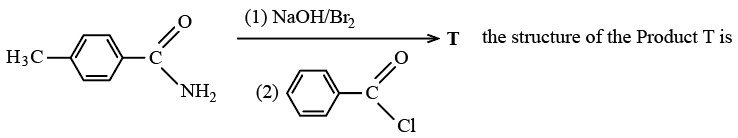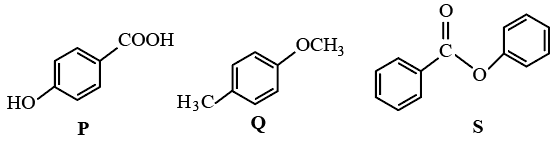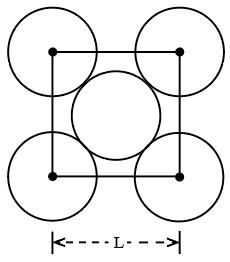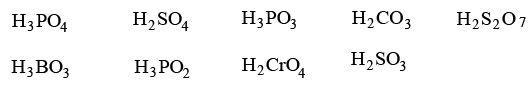Instructions

For the following questions answer them individually

Question 1

# The complex showing a spin-only magnetic moment of 2.82 B.M. isQuestion 2

# The species having pyramidal shape isQuestion 3

# In the reactionQuestion 4

# The compounds P, Q and Swere separately subjected to nitration using $$HNO_3/H_2SO_4$$ mixture. The major product formed in each case respectively, isQuestion 5

# The packing efficiency of the two-dimensional square unit cell shown below isQuestion 6

# Assuming that Hund's rule is violated, the bond order and magnetic nature of the diatomic molecule $$B_2$$ isQuestion 7

# The total number of diprotic acids among the following isBackspace
789
456
123
0.-
Clear AllQuestion 8

# Total number of geometrical isomers for the complex $$[RhCl(CO)(PPh_3)(NH_3)]$$ is

Backspace
789
456
123
0.-
Clear AllQuestion 9

# Among the following, the number of elements showing only one non-zero oxidation state isO, Cl, F, N, P, Sn. Tl, Na, Ti

Backspace
789
456
123
0.-
Clear AllQuestion 10

# Silver (atomic weight = $$108 g mol^{-1}$$) has a density of 10.5 g $$cm^{-3}$$. The number of silver atoms on a surface of area $$10^{-12} m^2$$ can be expressed in scientific notation as $$y \times 10^x$$. The value of x is

Backspace
789
456
123
0.-
Clear AllOR